# 熵模型—— 熵，条件熵，联合熵，相对熵，互信息及其关系，最大熵模型。。

 给定X的概率密度函数为fX(x), 若Y = aX, a是某正实数，求Y得概率密度函数fY(y).


1. 我们的假设应当满足全部已知条件；

2. 对未知的情况不做任何主观假设。

（一）熵

H(X) = -Σp(x)lnp(x)

p(xi) = 1/N, 则熵为：H(p) = -Σpi * lnpi = -Σ1/N * ln(1/N) = lnN

0 <= H(X) <= ln|X|

H(X, Y) - H(Y)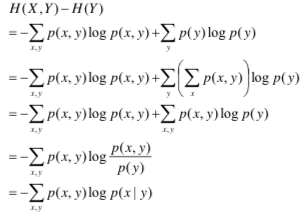（三）相对熵/交叉熵/K-L散度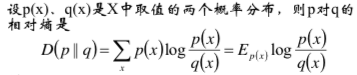D(p||q) >= 0, D(q||p) >= 0.

（四）互信息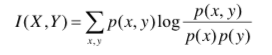H(X|Y) = H(X) - I(X, Y),

（五）各种熵之间的关系

H(X|Y) = H(X, Y) - H(Y); H(Y|X) = H(X, Y) - H(X) —— 条件熵的定义
H(X|Y) = H(X) - I(X, Y); H(Y|X) = H(Y) - I(X, Y)
I(X, Y) = H(X) - H(X|Y) = H(X) + H(Y) - H(X, Y) —— 也可以作为互信息的定义
H(X|Y) <= H(X)：
H(X)表示X的不确定度；H(X|Y)表示给定Y的情况下，X的不确定度。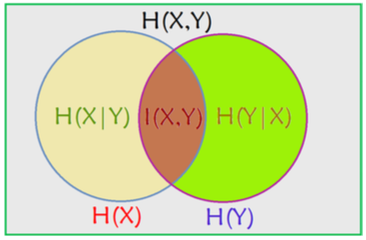（六）最大熵模型

1）最大熵的一般模型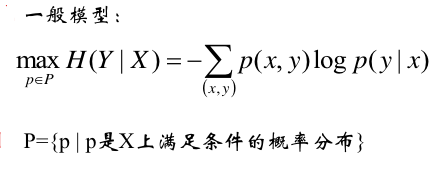2）Lagrange函数为：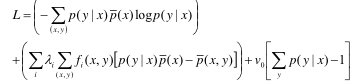p(x, y) = p(y | x) * p(x)，而p(x)是已知的，所以我们用p(x)_bar来表示已知量。

3）对p(y|x)求偏导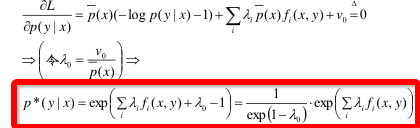4）归一化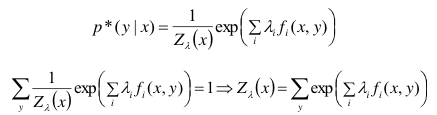5）与Logistic/SoftMax回归的对比

Logistic/SoftMax回归的后验概率形式：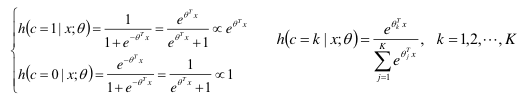Logistic回归是统计学习中的经典分类方法，可以用于二类分类也可以用于多类分类。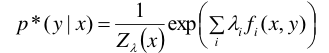Logistic回归模型与最大熵模型都属于对数线性模型。

【总结】